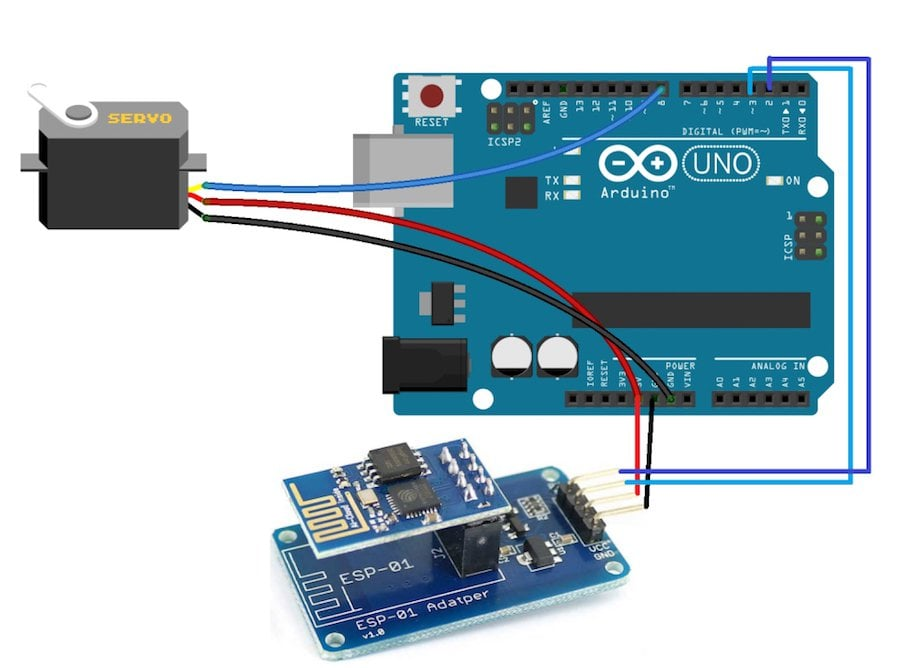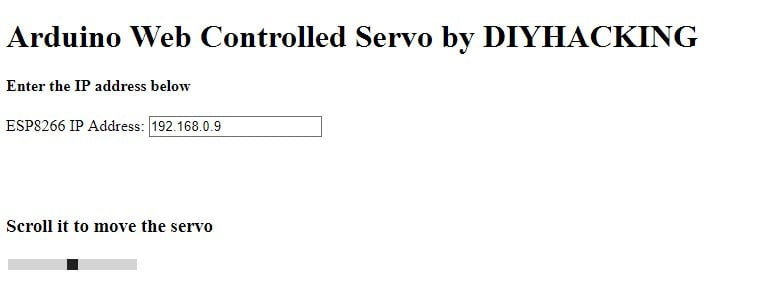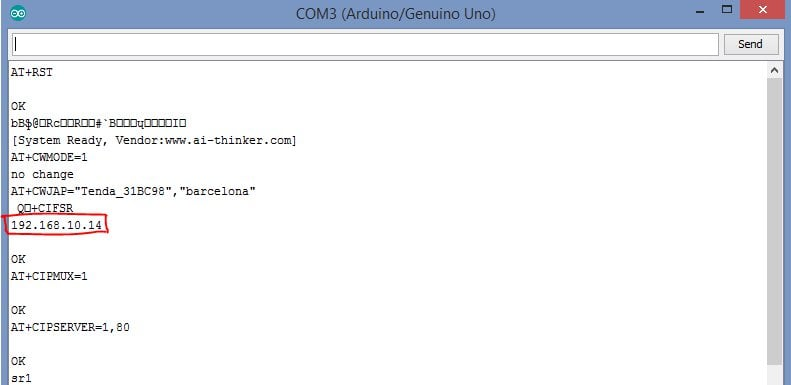Arduino

# How to Make a Web-Controlled Servo With Arduino and ESP8266March 23, 2018 by Muhammad Aqib
ShareUse an Arduino and an ESP8266 module to control a servo motor through a webpage.

In this project, we are going to control the servo motor through a webpage. The webpage will be created using an ESP8266 module and, by moving the slider on the webpage, the servo motor will move accordingly.

### Connecting the Arduino UNO to the ESP8266Web-controlled servo circuit diagram

First of all, connect the ESP8266 with Arduino. We have used an adapter to connect the esp8266 with the Arduino, which will make the connection much easier. The adapter has 5 to 3.3V regulator and you donâ€™t need to connect any external resistors with it.

• Connect the GND of adapter to the GND of Arduino
• Connect the VCC of adapter to the 5V of Arduino
• Connect the RX from the adapter to the pin 2 of Arduino
• Connect the TX pin from the adapter to the pin 3 of Arduino

After that, connect the servo motor with the Arduino. Make the connections of the servo motor with the Arduino as follows:

• Black wire of servo motor to the GND pin of Arduino
• Red wire of servo motor to the 5V pin of Arduino
• Yellow wire of servo motor to the pin 8 of Arduino

### Creating the Webpage

To control the servo motor through the webpage, we will have to make a webpage using the HTML language. The HTML code we created for our project can be downloaded from the end of this article. If you want to rename the file, then change the filename but make sure that it has â€œ.htmlâ€ at the end.

After that, download the JQUERY file (which is also given at the end of the article) and place this file in the same folder where you have placed the HTML file. After that, open the HTML and the webpage will look like this:Now, change the Wi-Fi name and password in the Arduino code with your Wi-Fi name and password. Then upload the code. Open the serial monitor and it will show you the IP address as shown in the figure below:Type this IP address in the space given on the webpage. Now, when you move the slider, the servo motor will move.

### Code

``````#include <SoftwareSerial.h>
#include <Servo.h>
SoftwareSerial esp8266(2,3);

#define DEBUG true
#define sg90_pin 8

Servo sg90;

int current_position = 170;
int vel = 10;
int minimum_position = 20;
int maximum_position = 160;

void setup()
{
sg90.attach(sg90_pin);
sg90.write(maximum_position);
sg90.detach();
Serial.begin(9600);
esp8266.begin(9600);

esp8266Data("AT+RST\r\n", 2000, DEBUG); //reset module
esp8266Data("AT+CWMODE=1\r\n", 1000, DEBUG); //set station mode
esp8266Data("AT+CWJAP=\"Tenda_31BC98\",\"barcelona\"\r\n", 2000, DEBUG);   //connect wifi network
while(!esp8266.find("OK")) { //wait for connection
}
esp8266Data("AT+CIFSR\r\n", 1000, DEBUG);
esp8266Data("AT+CIPMUX=1\r\n", 1000, DEBUG);
esp8266Data("AT+CIPSERVER=1,80\r\n", 1000, DEBUG);
}

void loop()
{
if (esp8266.available())
{
if (esp8266.find("+IPD,"))
{
String msg;
esp8266.find("?");
String command = msg.substring(0, 3);
String valueStr = msg.substring(4);
int value = valueStr.toInt();
if (DEBUG) {
Serial.println(command);
Serial.println(value);
}
delay(100);

//move servo1 to desired angle
if(command == "sr1") {
//limit input angle
if (value >= maximum_position) {
value = maximum_position;
}
if (value <= minimum_position) {
value = minimum_position;
}
sg90.attach(sg90_pin); //attach servo
while(current_position != value) {
if (current_position > value) {
current_position -= 1;
sg90.write(current_position);
delay(100/vel);
}
if (current_position < value) {
current_position += 1;
sg90.write(current_position);
delay(100/vel);
}
}
sg90.detach(); //dettach
}

}
}
}

String esp8266Data(String command, const int timeout, boolean debug)
{
String response = "";
esp8266.print(command);
long int time = millis();
while ( (time + timeout) > millis())
{
while (esp8266.available())
{
response += c;
}
}
if (debug)
{
Serial.print(response);
}
return response;
}``````

### Code Explanation

First of all, include the libraries for the software serial and for the servo. The software serial library will help us in using the TX and RX communication on other pins of the Arduino. The servo library will help us in moving the servo easily. After that, we defined the pins where we have connected the RX and TX from the ESP8266 and then we defined the pin where we attached the servo motor.

After that, we define the pins where we have connected the RX and TX from the ESP8266 and then we define the pin where we attached the servo motor.

``````#include <SoftwareSerial.h>
#include <Servo.h>
SoftwareSerial esp8266(2,3);
#define DEBUG true
#define sg90_pin 8``````

Then in the setup function, we tell the Arduino which pin we have connected the servo motor to and we moved the motor to the maximum position. Then we set the baud rate for the serial communication and the esp8266 at 9600. Set the baud rate of esp8266 according to your esp8266â€™s baud rate. Your esp8266 might have different baud rate.

Then we set the baud rate for the serial communication and the ESP8266 at 9600. You'll need to set the baud rate of ESP8266 according to your ESP8266â€™s baud rate. Your ESP8266 might have different baud rate.

``````sg90.attach(sg90_pin);
sg90.write(maximum_position);
sg90.detach();
Serial.begin(9600);
esp8266.begin(9600);``````

The following commands connect the ESP8266 to your Wi-Fi network and set the webserver at the IP address and port. It will show this in the serial monitor after uploading the code.

``````esp8266Data("AT+RST\r\n", 2000, DEBUG); //reset module
esp8266Data("AT+CWMODE=1\r\n", 1000, DEBUG); //set station mode
esp8266Data("AT+CWJAP=\"Tenda_31BC98\",\"barcelona\"\r\n", 2000, DEBUG);   //connect wifi network
while(!esp8266.find("OK")) { //wait for connection
}
esp8266Data("AT+CIFSR\r\n", 1000, DEBUG);
esp8266Data("AT+CIPMUX=1\r\n", 1000, DEBUG);
esp8266Data("AT+CIPSERVER=1,80\r\n", 1000, DEBUG);``````

The Arduino will see if the data is available or not. If the slider on the webpage is moved, then the ESP8266 sends the data to the Arduino according to the slider moved. The Arduino moves the servo motor according to the value given by the ESP8266.

``````if (esp8266.available())
{
if (esp8266.find("+IPD,"))
{
String msg;
esp8266.find("?");
String command = msg.substring(0, 3);
String valueStr = msg.substring(4);
int value = valueStr.toInt();``````

The following function sends the commands to the ESP8266 and will print the response of the ESP8266 on the serial monitor.

``````String esp8266Data(String command, const int timeout, boolean debug)
{
String response = "";
esp8266.print(command);
long int time = millis();
while ( (time + timeout) > millis())
{
while (esp8266.available())
{
response += c;
}
}``````

jquery.js webservo.html